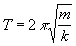# Derivation of the Simple Harmonic Motion Equation

This page shows how the equation (or rather proportionality) for the equation for the S.H.M. of a spring/trolley oscillator system can be derived. Its level is not meant to be above that expected at higher level GCSE/first year of A-Level.

The preliminary data are as follows:

Consider a trolley connected between two sets of springs of different spring constants. In both arrangements the trolley has the same mass and the same amplitude of oscillation is used. The period in (a) is twice that of (b) and therefore (b) oscillates at twice the speed of (a).

From this information we can see the following:

• At the point of rest, or the origin (the middle of the oscillation), when the trolleys are oscillating they have their highest velocity at this point.
• If we take the maximum displacement of the trolleys to be x, we can therefore say that in one complete oscillation both trolleys move 4x.
• From the displacement-time graphs (which were provided: they are sinusoidal) we can see that the period of the trolleys is the following:

Trolley (a) T

Trolley (b) T/2

(taking the period of (a) as being T, the period of (b) must be half).
• Therefore the average speeds of the trolleys are:

Trolley (a)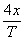Trolley (b)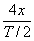From this we can say that Trolley (b) has twice the speed of (a).
• As the trolleys reach maximum speed when they travel to the midpoint of their oscillations, we can say that the time taken to reach this maximum speed is equal to one quarter of their respective periods (as in one complete oscillation the pass through the origin twice). Therefore the time taken for the trolleys to reach maximum velocity is:

Trolley (a) T/4

Trolley (b)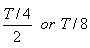• If we take the maximum velocity of the trolley (a) as being v, we can say that the maximum speed of trolley (b) is twice that of (a), as it covers the same distance in half the time. This is therefore 2v. From this we can calculate the average acceleration of the trolleys:

Trolley (a)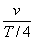Trolley (b)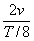Which can be simplified to give:

Trolley (a)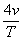Trolley (b)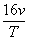Therefore (b) has 4 times the average acceleration of (a).

• From Newton's second Law, we know that F=ma, therefore as m is constant F is directly proportional to a. This then means that if a is increased four times, F is also increased by the same factor. We can therefore say that: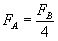(Where these are the forces acting on the respective trolleys).
• From Hooke's Law, we can see that as x, (the amplitude of oscillation) is constant, F is directly proportional to k, therefore if F is increased by a factor of 4, (as in the example above), k must have increased by the same factor. From this we can see that the spring in (b) must have four times the Hooke's constant of the spring in (a).
• Looking at what has been derived above, we can therefore see that the period of oscillation follows an inverse square law in relation to the Hooke's constant of the spring. This is because we can see that the period decreases as the Hooke's constant of the spring increases. Using the data provided, it can be seen that as k quadruples the period halves. This therefore suggests that: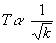• Looking at it in the light of acceleration, we can see that when this quadruples the period halves. Therefore, the relationship between period and acceleration is follows an inverse square law: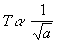• Using Newton's second Law, we can see that, if the mass stays constant the following is true: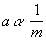• Therefore, if we substitute this into the proportionality connecting period with acceleration we find that: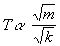• This relationship therefore connects period to mass and the Hooke's constant of the spring. To make it an equation, we must put in a constant. This constant is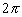. Hence: